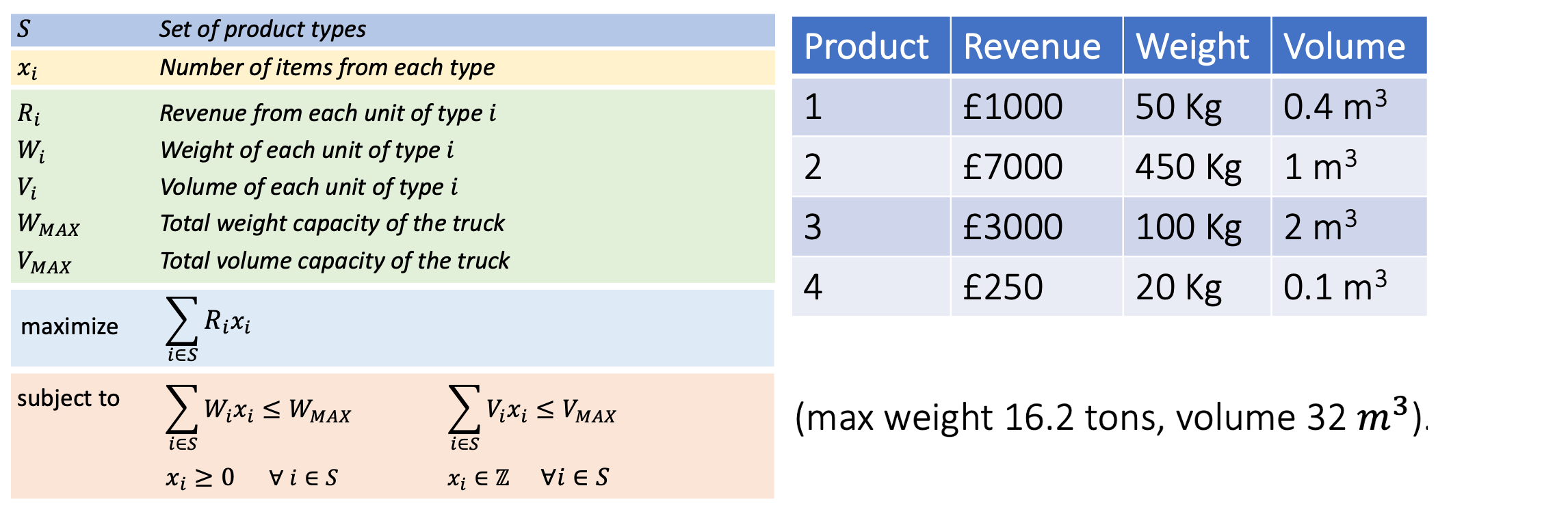# Notebook 3.7 - Knapsack Problem¶In [ ]:
# Setting up

from pulp import *

prob = LpProblem('prob', LpMaximize)


Note that we are now using LpMaximize, as we seek to maximize the value of our knapsack

In [ ]:
S = [str(i+1) for i in range(4)]

revenues = [1000, 7000, 3000, 250]
weights  = [  50,  450,  100,  20]
volumes  = [ 0.5,    1,    2, 0.1]

R = dict(zip(S, revenues))
W = dict(zip(S, weights))
V = dict(zip(S, volumes))

W_max = 16200
V_max = 32

In [ ]:
# Declaring decision variables.

x = LpVariable.dicts('x', S,  lowBound = 0, cat = LpInteger)

In [ ]:
# Declaring objectives

prob += lpSum([R[i]*x[i] for i in S])

for i in S:
prob += lpSum([W[i]*x[i] for i in S]) <= W_max
prob += lpSum([V[i]*x[i] for i in S]) <= V_max

In [ ]:
# Solving problem

prob.solve()

print('Selected items:\n')
for v in prob.variables():
print(f'  - Type {v.name.replace("x_","")} x {int(v.varValue):2}')

print('')

print('Total revenue:', prob.objective.value())


## Summary¶

from pulp import *

prob = LpProblem('prob', LpMaximize)

S = [str(i+1) for i in range(4)]

revenues = [1000, 7000, 3000, 250]
weights  = [  50,  450,  100,  20]
volumes  = [ 0.5,    1,    2, 0.1]

R = dict(zip(S, revenues))
W = dict(zip(S, weights))
V = dict(zip(S, volumes))

W_max = 16200
V_max = 32

x = LpVariable.dicts('x', S,  lowBound = 0, cat = LpInteger)

prob += lpSum([R[i]*x[i] for i in S])

for i in S:
prob += lpSum([W[i]*x[i] for i in S]) <= W_max
prob += lpSum([V[i]*x[i] for i in S]) <= V_max

prob.solve()

for v in prob.variables():
print(f'  - Type {v.name.replace("x_","")} x {int(v.varValue):2}')

print('')

print('Total Revenue:', prob.objective.value())